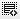## What is sharpe ratio and Standard Deviation0
Sharpe Ratio - All investments carry some kind of risk associated with them. Some investments have more risk, some have less risk. Its important that an investor can potentially get more return on a higher risk investment than a lower risk investment. Otherwise no one would invest in a risky security. So if someone says they got 30% return on an investment, it is meaningless unless you also know what risk was taken with that investment.

The Sharpe ratio helps the investor calculate the return he/she is getting in relation to the risk being taken. The higher the ratio the better. It is calculated as: (Asset's Return - Risk Free Return) / (Standard deviation of the assets returns).

The Standard deviation is used in this formula since it gives you a sense of how much the assets returns have varied from the mean historically. So this means that if you get a high return (numerator) with a low standard deviation (denominator) then the returns are good since the asset historically doesn't fluctuate and hence you have got a good return due to a smart investment.

Click on thisicon to add code snippet.